﻿ Effect of Thermal Gradient on Vibration of Non-Homogeneous Parallelogram Plate of Linearly Varying Thickness in Both Directions

### Effect of Thermal Gradient on Vibration of Non-Homogeneous Parallelogram Plate of Linearly Varying T...

Arun Kumar Gupta, Kumud Rana, Dharma Veer GuptaOPEN ACCESSPEER-REVIEWED

## Effect of Thermal Gradient on Vibration of Non-Homogeneous Parallelogram Plate of Linearly Varying Thickness in Both Directions

Arun Kumar Gupta1,, Kumud Rana2, Dharma Veer Gupta3

1Department of Mathematics, M.S. College, Saharanpur, U.P., India

2Department of Mathematics, Maharaja Agarsain Institute of Technology, Ghaziabad, U.P., India

3Department of Mathematics, College of Engineering Roorkee, Roorkee, U.A., India

### Abstract

The paper presented here is to study the effect of thermal gradient on vibration of non-homogeneous parallelogram plate of linearly varying thickness in both directions. Thermal induced vibration of non-homogeneous parallelogram plate has been taken as one dimensional temperature distribution in linear from only. For non-homogeneity of the plate material, density is assumed to vary linearly. Using the method of separation of variables; the governing differential equation is solved. An approximate but, quite convenient frequency equation is derived by Rayleigh-Ritz technique with two terms deflection function. The frequencies corresponding to the first two modes of vibration has been computed for a clamped parallelogram plate for different values of non -homogeneity constant, aspect ratio, thermal constant, thickness variation constant and skew angle.

• Gupta, Arun Kumar, Kumud Rana, and Dharma Veer Gupta. "Effect of Thermal Gradient on Vibration of Non-Homogeneous Parallelogram Plate of Linearly Varying Thickness in Both Directions." Journal of Mathematical Sciences and Applications 1.3 (2013): 43-49.
• Gupta, A. K. , Rana, K. , & Gupta, D. V. (2013). Effect of Thermal Gradient on Vibration of Non-Homogeneous Parallelogram Plate of Linearly Varying Thickness in Both Directions. Journal of Mathematical Sciences and Applications, 1(3), 43-49.
• Gupta, Arun Kumar, Kumud Rana, and Dharma Veer Gupta. "Effect of Thermal Gradient on Vibration of Non-Homogeneous Parallelogram Plate of Linearly Varying Thickness in Both Directions." Journal of Mathematical Sciences and Applications 1, no. 3 (2013): 43-49.

 Import into BibTeX Import into EndNote Import into RefMan Import into RefWorks

### 1. Introduction

A mathematical model is presented for the use of engineers, technocrats and research workers in space technology, mechanical sciences etc. which have to operate under elevated temperatures. Parallelogram plats with variable thickness are of great importance in a wide variety of engineering applications i.e. construction of wings, fins of rockets, missiles. As a result, the analysis of plate’s vibrations has attracted many research works, and has been considerably improved to achieve realistic results. As space technology has advanced, the need of the study of vibration of plates of certain aspect ratios with some simple restraints on the boundaries has also increased. The information for the first few modes of vibrations is essential for a constitution engineer before finalizing a design. Rapid strides in air- crafts, rockets and missiles have called for a deep insight in the dynamic response of elastic plates of variable thickness.

Gutierrez and Laura  have discussed the fundamental frequency of transverse vibration of rectangular, anisotropy plates of discontinuously variable thickness. Hearmen  solved the problem of the frequency of a flexural vibration of rectangular orthotropic plate with clamped and supported edges. Nair and Durvasula  studied the vibration of skew plate. Transverse vibration of trapezoidal plates of variable thickness has been discussed by Liew and Lam . Thermal effect on vibration of orthotropic rectangular plate with thickness variation is linearly & parabolically in x- and y- directions respectively studied by Gupta and Kumar . Kumud and Gupta  studied the thermal effect on vibration of non-homogeneous parallelogram plate of linearly varying thickness. Gupta and Kumud  discuss the thermal effect on vibration of non- homogeneous parallelogram plate of parabolically varying thickness. Thermal gradient effect on vibrations of non-homogeneous rectangular plate having continuously parabolically varying thickness in both directions is studied by Gupta et al. . Gupta et al.  studied the thermal effect on vibration of parallelogram plate of bi-directional linearly varying thickness.

In case of variable thickness plates, the governing differential equation of motion is found to have variable coefficient, this fact increase the difficulty to obtain the solution. According to the study of plates thermal field generate non-homogeneity in elastic bodies and the material properties are not constant but vary with the position in random manner. It is well known that in the presence of constant thermal gradient, the elastic coefficient of homogeneous materials become function of the space variable. Therefore, the aim of the present study is to determine the effect of linear thermal constant on free vibration of a clamped non- homogeneous parallelogram plate of linearly varying thickness in both directions. The Rayleigh-Ritz technique has been used to determine the frequency equations of the plate. The frequencies to the first two modes of vibration are obtained for a clamped parallelogram plate for various values non-homogeneity constant (α1), aspect ratio (a/b), thermal constant (α), taper constants (β1 & β2) and skew angle (θ).

### 2. The Transverse Equation of Motion

The parallelogram plate is assumed to be non-uniform, thin and isotropic. The skew co-ordinate system used, which are related as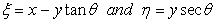(1)

The boundaries of the plate in skew co-ordinate are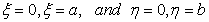(2)

For free vibration of the plate, the displacement function is periodic in time so it can expressed as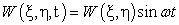(3)

where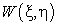is the maximum displacement at time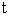and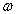is the angular frequency.

The maximum kinetic energy T and the strain energy V in the plate when it is executing transverse vibration mode shapeare :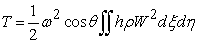(4)

and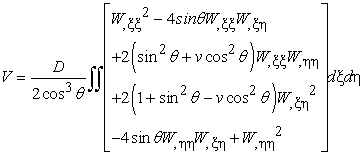(5)

A comma followed by a suffix denotes partial derivative with respect to that variable. Here D the flexural rigidity is given by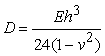(6)

Here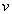is the Poisson Ratio.

### 3. Mathematical Analysis

Olsson  assumed that the parallelogram plate is subjected to a study one dimensional temperature distribution along the length, i.e. in ξ - direction.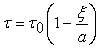(7)

Where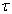denotes the temperature excess above the reference temperature at any point at a distance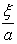and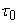at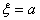.

The temperature dependence of the modulus of elasticity is given by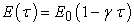(8)

where E0 is the value of Young modulus at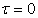.

Using equation (7) in (8), one obtains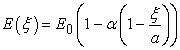(9)

where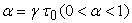, a parameter known as thermal constant.

The thickness variation of the plate is assumed to be parabolic in ξ direction only, therefore one has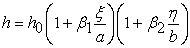(10)

where β1 and β2 are taper constants & h = h0 at ξ = 0.

Density of the plate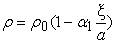(11)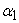is the non-homogeneity constant.

Using the equation (10) and (11) in equation (4) and (5), one gets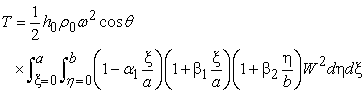(12)

and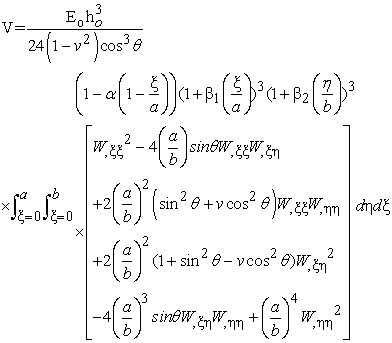(13)

### 4. Solution and Frequency Equation

Rayleigh-Ritz technique requires that the maximum strain energy must be equal to the maximum kinetic energy .It is therefore, necessary for the problem under consideration that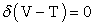(14)

for arbitrary variation of W satisfying relevant geometric boundary conditions.

For a parallelogram plate clamped along all four edges the boundary condition are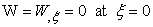, a and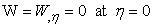, b and corresponding two term deflection is taken as: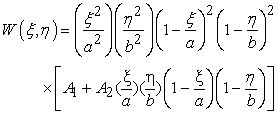(15)

Now equation (14) becomes after using (12) & (13)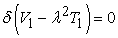(16)

where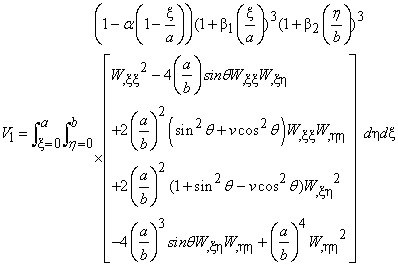(17)

and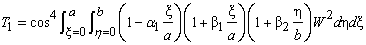(18)

here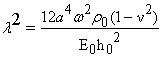(19)

is a frequency parameter.

Equation (16) involves the unknown A1 and A2 arising due to the substitution of W (ξ, η) from equation (15). These unknowns are to be determined from equation (16) for which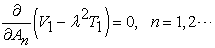(20)

The above equation simplifies to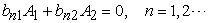(21)

For a non-trivial solution the determinant of the coefficients of equation (21) must be zero.

Therefore one gets the frequency equation as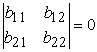(22)

From frequency equation (22) is quadratic equation in λ2 from which two values of λ can be found.

### 5. Results and Discussion

The frequency equation (22) is quadratic equation in λ2 from which two values of λ can be found. The frequency parameter λ corresponding to the first two modes of vibration of a clamped parallelogram plate has been computed for various values of non-homogeneity constant (α1), aspect ratio (a/b), thermal constant (α), taper constants (β1& β2) and skew angle (θ). The Poisson’s ratio (ν) is taken as 0.3. These results are summarized in Table 1 to Table 2.

Table 1 - Table 4 contains the value of frequency parameter of a clamped parallelogram plate for different values of thermal constant (α) for fixed value of aspect ratio (a/b) = 1.0 for the first two modes of vibration for taper constants (β1 = 0.4 & β2 = 0.6) and non-homogeneity constant (α1 = 0.0 & 0.4) and skew angle (θ). It has been seen from the tables that as thermal constant (α) increases, frequency parameter (λ) decrease and the effect of thermal constant on frequency parameter (λ) is more at angle 60° compare to 0°.

#### Table 4. Frequency parameter (λ) of a clamped parallelogram plate for different values of thermal constant (α), fixed aspect ratio a/b = 1.0 and θ = 60°

Table 5 - Table 8 gives the value of frequency parameter of a clamped parallelogram plate for different values of aspect ratio (a/b) and the combinations of α, β12 and α1 i.e. α = 0.0, β1 = 0.4, β2 = 0.6 and α1 = 0.0; α = 0.2, β1 = 0.4, β2 = 0.6 and α1 = 0.0; α = 0.0, β1 = 0.4, β2 = 0.6 and α1 = 0.4 and α = 0.2, β1 = 0.4, β2 = 0.6 and α1 = 0.4. It has been seen that as aspect ratio (a/b) increases, frequency parameter (λ) increases in all the cases for both modes of vibration. Also the effect of aspect ratio (a/b) on frequency parameter (λ) is increases as the angle increases.

#### Table 8. Frequency parameter (λ) of a clamped parallelogram plate for different values of aspect ratio a/b and α = 0.2, α1 = 0.4, β1 = 0.4, β2 = 0.6

Table 9 - Table 12 have the value of frequency parameter λ of a clamped parallelogram plate for different values of taper constant (β1) for fixed value of a/b = 1.0 and two values of β2 = 0.0 & 0.6 the combinations of α, and α1 i.e. α = 0.4, α1 = 0.0; α = 0.4, α1 = 0.4; α = 0.4, α1 = 0.0 and α = 0.4, α1 = 0.4. It has been seen from the tables that as taper constant (β1) increases, frequency parameter (λ) increase in all the cases for both modes of vibration. Also the effect of taper constant (β1) on frequency parameter (λ) is more at angle 60° compare to 45°, 30° & 0°.

#### Table 12. Frequency parameter (λ) of a clamped parallelogram plate for different values of taper constant (β1), fixed aspect ratio a/b = 1.0 and θ = 60°

Table 13 - Table 16 have the value of frequency parameter (λ) of a clamped parallelogram plate for different values of taper constant (β2) for fixed value of a/b = 1.0 and two values of β1 = (0.0 & 0.6) the combinations of α, and α1 i.e. α = 0.4, α1 = 0.0; α = 0.4, α1 = 0.4; α = 0.4, α1 = 0.0 and α = 0.4, α1 = 0.4. It has been seen that as taper constant (β2) increases frequency, parameter (λ) increase in all the cases for both modes of vibration. Also the effect of taper constant (β2) on frequency parameter (λ) is more at angle 60° compare to 45°, 30° & 0°.

#### Table 16. Frequency parameter (λ)of a clamped parallelogram plate for different values of taper constant (β2), fixed aspect ratio a/b = 1.0 and θ = 60°

Table 17-Table 20 contains the value of frequency parameter of a clamped parallelogram plate for different values of non-homogeneity constant (α1) for fixed value of aspect ratio (a/b) = 1.0 for the first two modes of vibration for taper constants (β1 = 0.4 & β2 = 0.6), thermal constant (α = 0.0 & 0.4) and skew angle (θ). It has been seen from the tables that as non-homogeneity constant (α1) increases, frequency parameter (λ) increases and also the effect of non-homogeneity constant (α1) on frequency parameter (λ) are more at angle 60° compare to 0°.

#### Table 20. Frequency parameter (λ) of a clamped parallelogram plate for different values of non-homogeneity constant (α1), fixed aspect ratio a/b = 1.0 and θ = 60°

Table 21 have the value of frequency parameter (λ) of a clamped parallelogram plate for different values of skew angle (θ) for fixed value of aspect ratio (a/b) = 1.0 for the first two modes of vibration for the combinations of α1 = 0.0, α = 0.0, β1 = 0.4 & β2 = 0.6; α1 = 0.4, α = 0.0, β1 = 0.4 & β2 = 0.6; α1 = 0.0,α = 0.2, β1 = 0.4 & β2 = 0.6; α1 = 0.4, α = 0.2, β1 = 0.4 & β2 = 0.6. It has been seen that as skew angle (θ) increases, frequency parameter (λ) increases in all the cases for both modes of vibration.

### 6. Conclusion

An analytical method is presented for free transverse vibration analysis of clamped non-homogeneous parallelogram plate of variable thickness with thermal gradient effect has been presented using Rayleigh-Ritz technique. Variation of thickness is taken as linearly in both directions while density and thermal effect taken as linearly in one direction i.e. along the length of the plate. It can be concluded that the vibration frequency parameter increases with increasing value of taper constants in both directions, aspect ratio, non-homogeneity constant and skew angle whereas it decreases with increasing value of thermal gradient. Also the effect of all the plate parameters is more pronounced for the higher modes than the lower.

### References

  Gutierrez, R.H. and Laura P.A.A. ‘Fundamental frequency of transverse vibration of rectangular, anisotropic plate of discontinuously varying thickness’, J. Sound and Vibration, Vol. 248, pp. 573-577, 2001. Hearmen, R.F.S.In article CrossRef  ‘The frequency of a flexural vibration of rectangular orthotropic plates with clamped or supported edges’, J. Appl. Mach., Trans. ASME, Vol. 26,No 4, pp. 537-540, 1959.In article  Nair, P.S. and Durvasula, S.‘Vibration of skew plates’, J. Sound and Vibration, Vol. 26, pp. 1-20, 1973.In article CrossRef  Liew, K. M. and Lim, M. K. ‘Transverse vibration of trapezoidal plates of variable thickness: symmetric trapezoids,’ J. Sound and Vibration, Vol. 165, No. 1, pp. 45-67, 1993.In article CrossRef  Gupta, A.K. and Kumar S. ‘Thermal effect on vibration of orthotropic rectangular plate with thickness variation is linearly & parabolically in x- and y- directions respectively, Journal of Mathematical Sciences and Applications , Vol. 1, No. 2, pp.32-35, 2013.In article  Kumud and Gupta, D.V. ’Thermal effect on vibration of non-homogeneous parallelogram plate of linearly varying thickness” , Journal of Experimental & Applied Mechanics, Vol. 4, No. 1, pp. 8-14, 2013.In article  Gupta, A.K. and Kumud ‘Thermal effect on vibration of non-homogeneous parallelogram plate of parabolically varying thickness’ Asian Journal of Applied Sciences, Vol. 1, No. 1, pp. 50-58, 2013.In article  Gupta, A.K., Panwar, V. and Vats, R.P. ‘Thermal gradient effect on vibrations of non-homogeneous rectangular plate having continuously parabolically varying thickness in both directions, Journal of Experimental & Applied Mechanics (2011), 2(1), 38-46(India).In article  Gupta, A.K., Kumar, M., Kumar, S. and Khanna, A.‘Thermal effect on vibration of parallelogram plate of bi-directional linearly varying thickness, Applied Mathematics (2011), 2(1), 33-38(USA).In article CrossRef  Leissa, A.W. ‘Vibration of plate’, NASA SP-160, U.S. Govt. Printing office, 1969.In article  Leissa, A.W. ‘Recent studies in plate vibration 1981-1985: Part II, complicating effects’, The shock and Vibration Digest., Vol. 19, pp.10-24, 1987.In article CrossRef  Olsson, U. ‘On free vibrations at temperature dependent material properties and transient temperature fields’, J. Appl. Mach., Trans. ASME, Vol. 39, 723-726, 1972.In article CrossRef